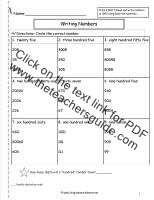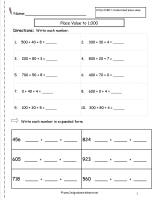# Reading and Writing Numbers to 1000 Worksheets

## Reading Numbers to 1000 Worksheets, Writing Numbers to 1000 Worksheets

###Reading Numbers to 1000

Common Core State Standards: CCSS 2.NBT.3 Read and write numbers to 1000 using base ten numberals....
Students read number words and circle the correct number.

###Writing Numbers Worksheet

Common Core State Standards: CCSS 2.NBT.3 Read and write numbers to 1000 using base ten numberals....
Students write two digit numbers in expanded form. 2 digits.

###Writing Numbers Worksheet

Common Core State Standards: CCSS 2.NBT.3 Read and write numbers to 1000 using base ten numberals....
Students write the numbers in expanded form. 3 digits.

###Writing Numbers Worksheet

Common Core State Standards: CCSS 2.NBT.3 Read and write numbers to 1000 using base ten numberals....
Students write the number in expanded and standard form.

###Writing Numbers Worksheet

Common Core State Standards: CCSS 2.NBT.3 Read and write numbers to 1000 using base ten numberals....
Students write the number in expanded and standard form.